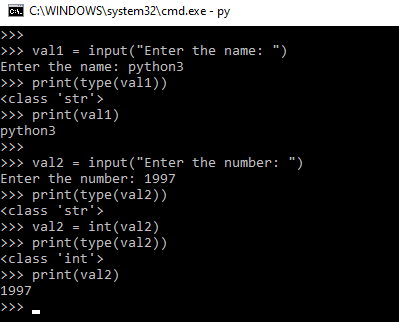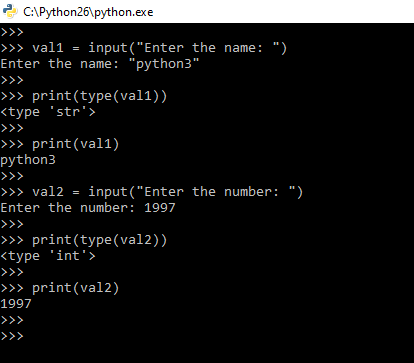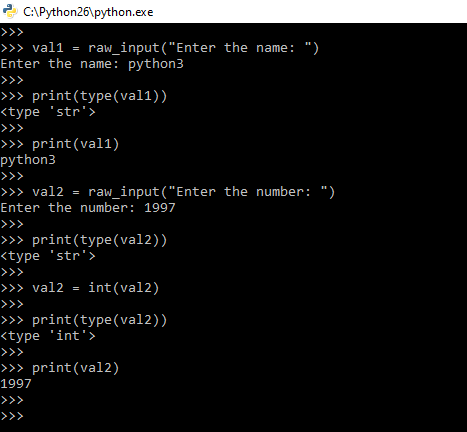# Difference between input() and raw_input() functions in Python

Developers often have a need to interact with users, either to get data or to provide some sort of result. Most programs today use a dialog box as a way of asking the user to provide some type of input. While Python provides us with two inbuilt functions to read the input from the keyboard.

## input() function

Python `input() `function is used to take the values from the user. This function is called to tell the program to stop and wait for the user to input the values. It is a built-in function. The `input() `function is used in both the version of Python 2.x and Python 3.x. In Python 3.x, the input function explicitly converts the input you give to type string. But Python 2.x input function takes the value and type of the input you enter as it is without modifying the type.

Example program in Python3

 `# Python program to demonstrate ` `# input() function in Python3.x ` ` `  ` `  `val1 ``=` `input``(``"Enter the name: "``) ` ` `  `# print the type of input value ` `print``(``type``(val1)) ` `print``(val1) ` ` `  ` `  `val2 ``=` `input``(``"Enter the number: "``) ` `print``(``type``(val2)) ` ` `  `val2 ``=` `int``(val2) ` `print``(``type``(val2)) ` `print``(val2) `

Input and OutputHere, the value “python3” take from the user and store it in the val1 variable. The type of the value stored is always string for input function only for Python 3.x. The value “1997” take from the user and store it in the variable val2. Now, the type of variable val2 is a string and we have to convert the type to an integer using `int()` function. The val2 variable stores the value “1997” as an integer type.

Example program in Python2

 `# Python program to demonstrate ` `# input() function in Python2.x ` ` `  ` `  `val1 ``=` `input``(``"Enter the name: "``) ` `print``(``type``(val1)) ` `print``(val1) ` ` `  `val2 ``=` `input``(``"Enter the number: "``) ` `print``(``type``(val2)) ` `print``(val2) `

Input and OutputHere, the value “python3” take from the user and store it in the val1 variable. The function takes the value and type of the input you enter as it is without modifying the type. The type of value in val1 is string type. The value “1997” takes from the user and store it in the variable val2. Now, the type of variable val2 is integer type. We don’t need to explicitly change the variable type.

## raw_input() function

Python raw_input function is used to get the values from the user. We call this function to tell the program to stop and wait for the user to input the values. It is a built-in function. The input function is used only in Python 2.x version. The Python 2.x has two functions to take the value from the user. The first one is input function and another one is `raw_input() `function. The `raw_input() `function is similar to `input() `function in Python 3.x. Developers are recommended to use raw_input function in Python 2.x. Because there is a vulnerability in input function in Python 2.x version.

Example program in Python2

 `# Python program to demonstrate ` `# input() function in Python2.x ` ` `  ` `  `val1 ``=` `raw_input``(``"Enter the name: "``) ` `print``(``type``(val1)) ` `print``(val1) ` ` `  `val2 ``=` `raw_input``(``"Enter the number: "``) ` `print``(``type``(val2)) ` `val2 ``=` `int``(val2) ` `print``(``type``(val2)) ` `print``(val2) `

Input and OutputHere, the value “python3” take from the user and store it in the val1 variable. The type of the value stored is always string for raw_input function. The value “1997” take from the user and store it in the variable val2. Now, the type of variable val2 is a string and we have to convert the type to an integer using` int()` function. The val2 variable stores the value “1997” as an integer type.

My Personal Notes arrow_drop_upCheck out this Author's contributed articles.

If you like GeeksforGeeks and would like to contribute, you can also write an article using contribute.geeksforgeeks.org or mail your article to contribute@geeksforgeeks.org. See your article appearing on the GeeksforGeeks main page and help other Geeks.

Please Improve this article if you find anything incorrect by clicking on the "Improve Article" button below.